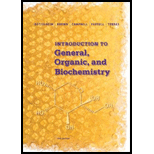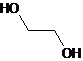# 14-8 Answer true or false. The functional group of an alcohol is the —OH (hydroxyl) group. The parent name of an alcohol is the name of the longest carbon chain that contains the —OH group. A primary alcohol contains one —OH group, and a tertiary alcohol contains three —OH groups. In the 1UPAC system, the presence of three —OH groups is shown by the ending —trial. A glycol is a compound that contains two —OH groups. The simplest glycol is ethylene glycol, HOCH 2 CH 2 OH. Because of the presence of an —OH group, all alcohols are polar compounds. The boiling points of alcohols increase with increasing molecular weight. (h, The solubility of alcohols in water increases with increasing molecular weight.### Introduction to General, Organic a...

11th Edition
Frederick A. Bettelheim + 4 others
Publisher: Cengage Learning
ISBN: 9781285869759### Introduction to General, Organic a...

11th Edition
Frederick A. Bettelheim + 4 others
Publisher: Cengage Learning
ISBN: 9781285869759

#### Solutions

Chapter 14, Problem 14.8P
Textbook Problem

## 14-8 Answer true or false. The functional group of an alcohol is the —OH (hydroxyl) group. The parent name of an alcohol is the name of the longest carbon chain that contains the —OH group.A primary alcohol contains one —OH group, and a tertiary alcohol contains three —OH groups.In the 1UPAC system, the presence of three —OH groups is shown by the ending —trial.A glycol is a compound that contains two —OH groups. The simplest glycol is ethylene glycol, HOCH2CH2OH.Because of the presence of an —OH group, all alcohols are polar compounds.The boiling points of alcohols increase with increasing molecular weight.(h, The solubility of alcohols in water increases with increasing molecular weight.

Expert Solution
Interpretation Introduction

(a)

Interpretation:

To analyse whether the given statement: The functional group of an alcohol is the −OH (hydroxyl) group, is true or not.

Concept Introduction:

Alcohols are those organic compounds wherein a hydrogen atom of the aliphatic carbon molecule is substituted by a hydroxyl group. Hence an alcohol molecule has two parts, one comprising the alkyl group and the other carrying hydroxyl group.

The boiling point of alcohols is significantly higher than those of alkanes having similar molecular weights. The solubility of alcohol is inversely proportional to the size of the alkyl group. Alcohols reacts with active metals like sodium, potassium and many more to form the corresponding alkoxide.

The functional group of an alcohol is the −OH (hydroxyl) group. Thus, the statement is true.

### Explanation of Solution

The functional group of any alcohol is the —OH group. Therefore, the given statement is true.

Expert Solution
Interpretation Introduction

(b)

Interpretation:

To analyse whether the given statement: The parent name of an alcohol is the name of the longest carbon chain that contains the −OH group, is true or not.

Concept Introduction:

Alcohols are those organic compounds wherein a hydrogen atom of the aliphatic carbon molecule is substituted by a hydroxyl group. Hence an alcohol molecule has two parts, one comprising the alkyl group and the other carrying hydroxyl group.

The boiling point of alcohols is significantly higher than those of alkanes having similar molecular weights. The solubility of alcohol is inversely proportional to the size of the alkyl group. Alcohols reacts with active metals like sodium, potassium and many more to form the corresponding alkoxide.

The parent name of an alcohol is the name of the longest carbon chain that contains the −OH group.Thus, the statement is true.

### Explanation of Solution

According to IUPAC nomenclature, the parent name of an alcohol is the name of the longest.

carbon chain that contains the —OH group. Therefore, the given statement is true.

Expert Solution
Interpretation Introduction

(c)

Interpretation:

To analyse whether the given statement: A primary alcohol contains one −OH group, and a tertiary alcohol contains three-OH groups, is true or not.

Concept Introduction:

Alcohols are those organic compounds wherein a hydrogen atom of the aliphatic carbon molecule is substituted by a hydroxyl group. Hence an alcohol molecule has two parts, one comprising the alkyl group and the other carrying hydroxyl group.

The boiling point of alcohols is significantly higher than those of alkanes having similar molecular weights. The solubility of alcohol is inversely proportional to the size of the alkyl group. Alcohols reacts with active metals like sodium, potassium and many more to form the corresponding alkoxide.

A primary, secondary, and tertiary alcohol contains only one -OH groups but the connectivity of carbon atoms bearing −OH group is different. Thus, the statement is false.

### Explanation of Solution

Primary alcohol is alcohol in which the -OH group is linked to carbon that is connected to only one carbon atom. It has like -CH2 OH group in molecule. Secondary alcohol is alcohol in which the -OH group is linked to carbon that is connected to two other carbon atoms. Tertiary alcohol is alcohol in which the -OH group is linked to carbon that is connected to three other carbon atoms. Primary, secondary, and tertiary alcohols have only one -OH group in their molecule but the connectivity of carbon atom bearing -OH group is different. Therefore, the given statement is false.

Expert Solution
Interpretation Introduction

(d)

Interpretation:

To analyse whether the given statement: In the IUPAC system, the presence of three −OH groups is shown by the ending −triol, is true or not.

Concept Introduction:

Alcohols are those organic compounds wherein a hydrogen atom of the aliphatic carbon molecule is substituted by a hydroxyl group. Hence an alcohol molecule has two parts, one comprising the alkyl group and the other carrying hydroxyl group.

The boiling point of alcohols is significantly higher than those of alkanes having similar molecular weights. The solubility of alcohol is inversely proportional to the size of the alkyl group. Alcohols reacts with active metals like sodium, potassium and many more to form the corresponding alkoxide.

In the IUPAC system, the presence of three −OH groups is shown by the ending −triol. Thus, the statement is true.

### Explanation of Solution

In IUPAC nomenclature, the presence of three -OH groups is shown by the ending triol Therefore, the given statement is true.

Expert Solution
Interpretation Introduction

(e)

Interpretation:

To analyze whether the given statement: A glycol is a compound that contains two-OH groups. The simplest glycol is ethylene glycol, HOCH2CH2OH, is true or not.

Concept Introduction:

Alcohols are those organic compounds wherein a hydrogen atom of the aliphatic carbon molecule is substituted by a hydroxyl group. Hence an alcohol molecule has two parts, one comprising the alkyl group and the other carrying hydroxyl group.

The boiling point of alcohols is significantly higher than those of alkanes having similar molecular weights. The solubility of alcohol is inversely proportional to the size of the alkyl group. Alcohols reacts with active metals like sodium, potassium and many more to form the corresponding alkoxide.

A glycol is a compound that contains two-OH groups. The simplest glycol is ethylene glycol, HOCH2CH2OH.Thus, the statement is true.

### Explanation of Solution

Glycol is a compound that has two -OH groups attached to carbon atoms. Ethyl glycol is the simplest form of glycol. Its structural formula is:Therefore, the given statement is true.

Expert Solution
Interpretation Introduction

(f)

Interpretation:

To analyze whether the given statement: Because of the presence of an-OH group, all alcohols are polar compounds, is true or not.

Concept Introduction:

Alcohols are those organic compounds wherein a hydrogen atom of the aliphatic carbon molecule is substituted by a hydroxyl group. Hence an alcohol molecule has two parts, one comprising the alkyl group and the other carrying hydroxyl group.

The boiling point of alcohols is significantly higher than those of alkanes having similar molecular weights. The solubility of alcohol is inversely proportional to the size of the alkyl group. Alcohols reacts with active metals like sodium, potassium and many more to form the corresponding alkoxide.

Because of the presence of an -OH group, all alcohols are polar compounds. Thus, the statement is true.

### Explanation of Solution

Polar molecules have polar covalent bonds connected between the atoms. Methanol is an example of alcohol. Here, the O-C bond and O-H bond are polar covalent bonds. In methanol, there is a difference in electronegativity between the O-C bond (3.5-2.5 = 1.0) and O-H bond (3.5-2.1 = 1.4) Electronegativity is the tendency of an atom to attract electrons towards itself. Due to the presence of the O-H bond, all alcohols are polar molecules.

Therefore, the given statement is true.

Expert Solution
Interpretation Introduction

(g)

Interpretation:

To analyze whether the given statement: The boiling point of alcohols increases with increasing molecular weight, is true or not.

Concept Introduction:

Alcohols are those organic compounds wherein a hydrogen atom of the aliphatic carbon molecule is substituted by a hydroxyl group. Hence an alcohol molecule has two parts, one comprising the alkyl group and the other carrying hydroxyl group.

The boiling point of alcohols is significantly higher than those of alkanes having similar molecular weights. The solubility of alcohol is inversely proportional to the size of the alkyl group. Alcohols reacts with active metals like sodium, potassium and many more to form the corresponding alkoxide.

The boiling point of alcohols increases with increasing molecular weight. Thus, the statement is true.

### Explanation of Solution

The temperature at which the atmospheric pressure is equal to the vapor pressure is known as boiling point. As the molecular weight increases, more atoms are involved in the non-covalent interaction. Due to this tact, more energy (more temperature) is required to break this non-covalent interactions.Therefore, the given statement is true.

Expert Solution
Interpretation Introduction

(h)

Interpretation:

To analyze whether the given statement: The solubility of alcohols in water increases with increasing molecular weight, is true or not.

Concept Introduction:

Alcohols are those organic compounds wherein a hydrogen atom of the aliphatic carbon molecule is substituted by a hydroxyl group. Hence an alcohol molecule has two parts, one comprising the alkyl group and the other carrying hydroxyl group.

The boiling point of alcohols is significantly higher than those of alkanes having similar molecular weights. The solubility of alcohol is inversely proportional to the size of the alkyl group. Alcohols reacts with active metals like sodium, potassium and many more to form the corresponding alkoxide.

The solubility of alcohols in water decreases with increasing molecular weight. Thus, the statement is false.

### Explanation of Solution

Alcohols are more soluble in water than hydrocarbons. This is due to the presence of the O-H group in alcohol. The O-H group undergoes hydrogen bonding with a hydrogen atom in the water molecule.

As the molecular weight increases, simultaneously the hydrocarbon chain in alcohol will also increase. Now the alcohol stars behaving more like hydrocarbons. Therefore, the solubility decreases with the increase in molecular weight.

Therefore, the given statement is false.

### Want to see more full solutions like this?

Subscribe now to access step-by-step solutions to millions of textbook problems written by subject matter experts!

### Want to see more full solutions like this?

Subscribe now to access step-by-step solutions to millions of textbook problems written by subject matter experts!